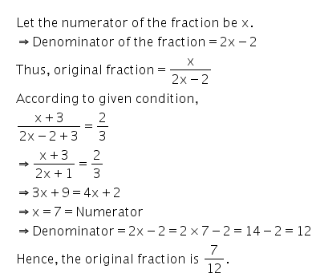# In a fraction twice the numerator is 2 more than the denominator

In a fraction, twice the numerator is 2 more than the denominator. If 3 is added to the numerator and the denominator, the new fraction is 2/3. Find the original fraction.